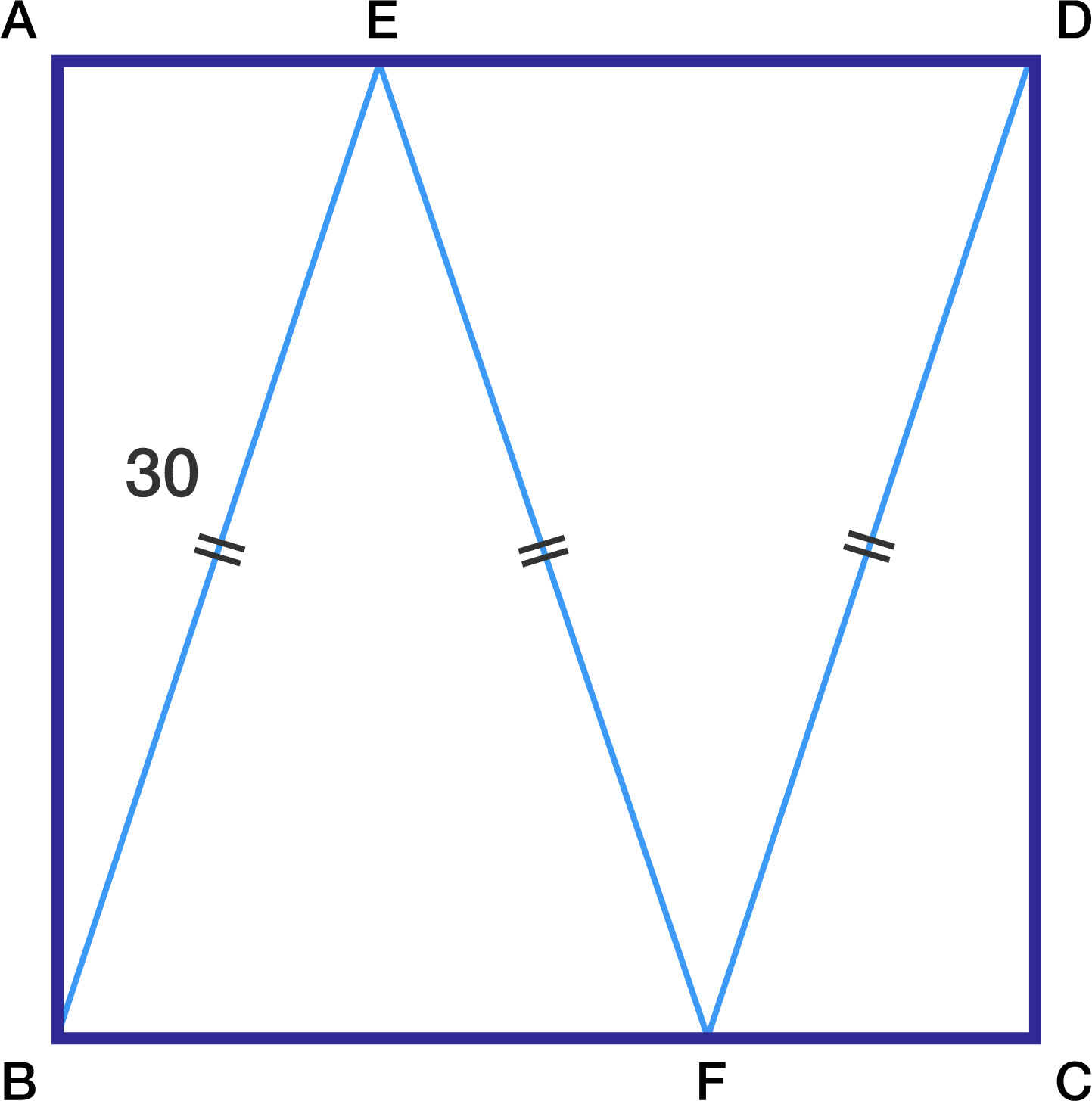# Tricky Treat!

Geometry Level 2On the square $ABCD$, point $E$ lies on the side $AD$ and $F$ lies on $BC$ so that $BE = EF = FD = 30 \text{ cm}$. What is the area of the square (in $\text{cm}^2$)?

×